# 电路布线问题（分支限界法）

### 一.问题描述

• 印刷电路板将布线区域划分成n*m个方格阵列。
• 精确的电路布线问题要求确定连接方格a的中点到方格b的中点的最短布线方案。
• 在布线时，电路只能沿直线或直角布线。
• 为了避免线路相交，已布了线的方格做了封锁标记，其他线路不允许穿过被封锁的方格。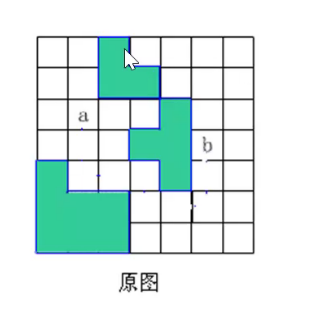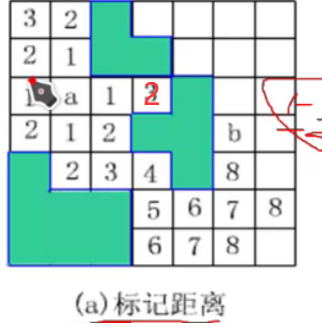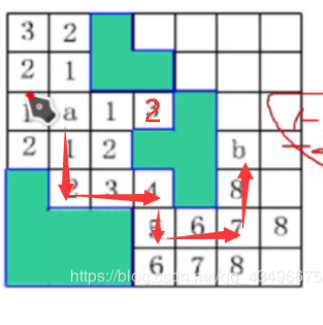### 二.算法设计与分析

1.回溯vs分支限界

①深度优先：搜索到可行解不一定是最优解，需要将所有可行解搜索出来进行比较。

②广度优先：一层一层搜索，只要搜索到可行解那么必定是最优解，进入树的深度就是路径的长度。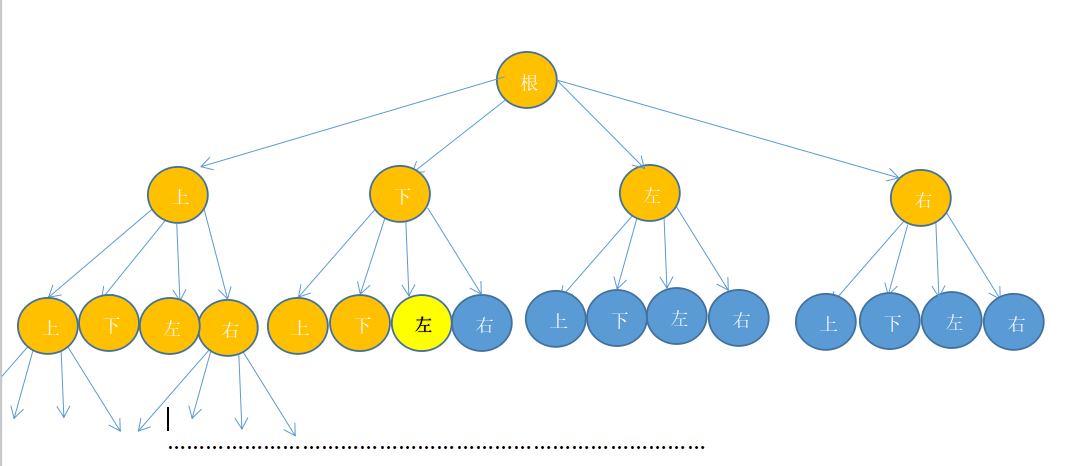2.过程：

• 从起始位置a开始将它作为第一个扩展结点
• 与该结点相邻并且可达的方格被加入到活缓点队列中，并且将这些方格标记为1
• 接着从活结点队列中取出队首作为下一个扩展结点，并将与当前扩展结点相邻且未标记过的方格标记为2，并存入活节点队列
• 这个过程一直继续到算法搜索到目标方格b或活结点队列为空时为止(表示没有通路)

3.算法实现

①定义小方格位置

• 定义一个表示电路板上小方格位置的类Position,它的两个私有成员row和col分别表示小方格所在的行和列。
• 在电路板的任何一个方格处，布线可沿右、下、左、上4个方向进行。沿这4个方向的移动分别记为0,1，2，3。沿着这4个方向前进一步相对于当前方格的位移如下表所示。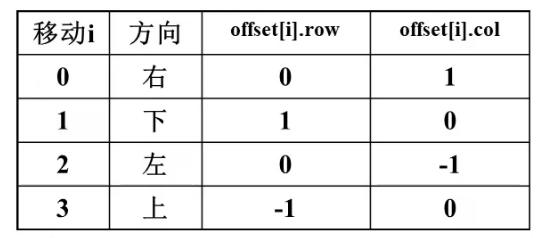②实现方格阵列

• 用二维数组grid表示所给的方格阵列。初始时，grid[i][j]=0，表示该方格允许布线，而grid[i][j]=1表示该方格被封锁，不允许布线。
• 为了便于处理方格边界的情况，算法在所给方格阵列四周设置一圈标记为“1”的附加方格，即可识别方阵边界。

③初始化

• 算法开始时，测试初始方格与目标方格是否相同。如果相同，则不必计算，直接放回最短距离0，否则算法设置方格阵列的边界，初始化位移矩阵offset。

④算法搜索步骤

• 算法从start开始，标记所有标记距离为1的方格并存入活结点队列，然后依次标记所有标记距离为2,3.....的方格，直到到达目标方格finish或活结点队列为空时为止
#include <iostream>
#include <queue>
using namespace std;
int m=8;
int n=8;
int grid;
int indexcount=0;
struct Position{
int row;
int col;
};

bool FindPath(Position start, Position finish, int & PathLen, Position &path){//计算从起点位置start到目标位置finish的最短布线路径，找到最短布线路径则返回true，否则返回false
if((start.row==finish.row) & & (start.col==finish.col)){
PathLen=0;
cout<<"start=finish"<<endl;
return true;
} //start=finish
//设置方格阵列“围墙”初始化图，-1为未访问
for(int i=1; i<9; i++){
for(int j=1; j<9; j++)
grid[i][j]=-1;
}
//添加阻挡点
grid=-2;
for(int i=0; i<= m+1; i++)
grid[i]=grid[n+1][i]=-2; //顶部和底部.
for(int i=0; i<= n+1; i++)
grid[i]=grid[i][m+1]= 2; //左翼和右翼
//初始化相对位移
cout<<"完整图"<<endl;
showPath( );
Position offset;
offset.row=0; offset.col=1; //右
offset.row=1; offset.col=0; //下
offset.row=0; offset.col=-1;//左
offset.row=-1;offset.col=0; //上
int NumOfNbrs=4;//相邻方格数
Position here;
here.row=start.row;
here.col=start.col;
grid[start.row][start.col]=0;
//标记可达方格位置
cout<<"布线前图"<<endl;
showPath( );
queue<Position> Q;
do //标记相邻可达方格
{
for(int I=0; I<NumOfNbrs; I++)
{
nbr.row=here.row + offset[I].row;
nbr.col=here.col + offset[I].col;
if(grid[nbr.row][nbr.col]==-1) //该方格未被标记
{
grid[nbr.row][nbr.col=grid[here.row][here.col]+1;
if(nbr.row== finish.row) & &(nbr.col==finish.col)) break; //完成
Q.push(nbr); .
}
//是否到达目标位置finish?
if(nbr.row ==finish.row)& &(nbr.col == finish.col) break;//完 成
//活结点队列是否非空?
if(Q.emptyO) return false;//无解
here = Q.front( );
Q.pop( );//取下一个扩展结点
indexcount++;
} while(true);
//构造最短布线路径
PathLen=grid[finish.row][finish.col];
path=new Position[PathLen];
//从目标位置finish开始向起始位置回溯
here =finish;
for(int j=PathLen-1; j>=0; j--)
path[j]=here;
//找前驱位置
for(int i=0; i<NumOfNbrs; i++)
{
nbr.row=here.row+offset[i].row;
nbr.col=here.col+offset[i].col;
if(grid[nbr.row][nbr.col]== j)
{
break;
}
here=nbr;//向前移动
return PathLen;
}


### 三.低效率的回溯法的思路：

①解向量（x1,x2……xn) n表示可行解的路径长度，其中xi表示第i步走的方向

②解空间：子集树

③约束函数：不能进入边界或者已经标记过的方格

④记录当前最优解的路径长度bestl，遇到可行解，与最优解比较

05-2211-18
03-27
06-243962
05-251226
10-102283
08-205776
05-101万+
10-19605
©️2020 CSDN 皮肤主题: 终极编程指南 设计师:CSDN官方博客